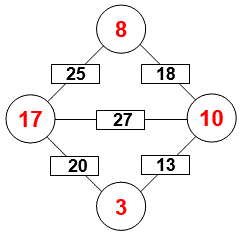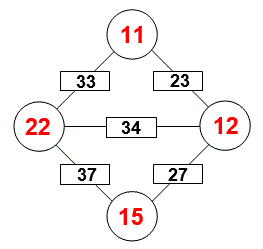Select Page

Each month, a new set of puzzles will be posted.  Come back next month for the solutions and a new set of puzzles, or subscribe to have them sent directly to you.

## RELATIONAL Challenge

In the following puzzle, there is a common arithmetic relationship between the set of equations that allow their respective solutions as shown. Determine the relationship to find the unknown relational value (?).

1 + 4 = 5
2 + 5 = 11
3 + 6 = 19
5 + 8 = ?

## MIND-Xpander (Circular Logic Challenge – Level 1)

You have 50 sticks standing upright in a circle (#1 to #50). Starting with stick #1, knock over the next stick (#2) in the circle. Then continue by knocking over every second stick (next is #4). Keep going around and around the circle until one stick remains standing. What number is the last stick standing?

What if you start with stick #4 and continue in same manner as above?

## Feedback

There are more than one way of doing these puzzles and may well be more than one answer.  Please let me and others know what alternatives you find by commenting below.  We also welcome general comments on the subject and any feedback you'd like to give.

If you have a question that needs a response from me or you would like to contact me privately, please use the contact form.

## Get more puzzles!

If you've enjoyed doing the puzzles, consider ordering the books;

• Book One - 150+ of the best puzzles
• Book Two - 200+ with new originals and more of your favourites

Both in a handy pocket sized format.   Click here for full details.

## IND-Xpander puzzle

In a class of 78 students, 41 are taking French and 22 are taking German. Of the students taking French and German, 9 are taking both courses. How many students are not enrolled in either course?

Solution

78 = (41 – 9) + (22 – 9) + 9 + (neither)
(neither) = 78 – 32 – 13 – 9 = 24 students

You cash your paycheck and save half of it. With the rest, you pay a debt of \$20 and buy something for \$5. Then spending 1/5 of the remaining money, you have \$12 left. How much was your paycheck?

Solution

Let x = total pay check, then

x = 1/2x + 20 + 5 + 1/5 (1/2x – 25) + 12
x = 1/2x + 25 + 1/10x – 5 + 12
x – 1/2x – 1/10x = 32
4/10x = 32  and therefore, x = \$80

In the Arithmagon, the value in each box is the sum of the values in the circles on each side of it. Determine the value in each circle.## EQUATE+2 Puzzle

Each row, column & diagonal is an equation and you use the numbers 1 to 9 to complete the equations. Each number can be used only once. ‘Two’ numbers have been provided to get you started. Find the remaining eight numbers that satisfies all the resulting equations. Note: multiplication (x) & division (/) are performed before addition (+) and subtraction (-).